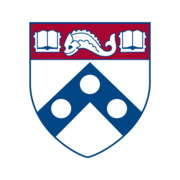このコースについて

67,174 最近の表示

スケジュールに従って期限をリセットします。

100%オンライン

High school or college math.

“Introduction to Python Programming” Coursera course or equivalent prior knowledge of introductory Python.

学習内容

• Apply basic data science techniques using Python

• Understand and apply core concepts like Data Frames and joining data, and use data analysis libraries like pandas, numpy, and matplotlib

• Demonstrate how to load, inspect, and query real-world data, and answer basic questions about that data

• Analyze data further by applying learned skills in data aggregation and summarization, as well as basic data visualization

習得するスキル

• Data Science
• Python Libraries
• Python Programming
• Data Analysis
• Data Visualization (DataViz)

スケジュールに従って期限をリセットします。

100%オンライン

High school or college math.

“Introduction to Python Programming” Coursera course or equivalent prior knowledge of introductory Python.

提供：1

6時間で修了

Module 1 : Loading, Querying, & Filtering Data Using the csv Module

6時間で修了
8件のビデオ （合計31分）, 9 学習用教材, 3 個のテスト
2

週 2

5時間で修了

5時間で修了
14件のビデオ （合計31分）, 5 学習用教材, 4 個のテスト
3

5時間で修了

Module 3 : Summarizing & Visualizing Data

5時間で修了
18件のビデオ （合計22分）, 5 学習用教材, 4 個のテスト

Introduction to Programming with Python and Java専門講座についてよくある質問

さらに質問がある場合は、受講者ヘルプセンターにアクセスしてください。